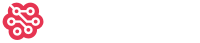## Users by rep bracket

3

(click on this box to dismiss)Q&A for users and developers of hardware and software for Raspberry Pi

``````-- Users by rep bracket

select
count(CASE WHEN Reputation = 1 THEN 1 ELSE NULL END ) as 'rep = 1',
count(CASE WHEN (Reputation > 1 and Reputation < 10) THEN 1 ELSE NULL END ) as '1 < rep < 9',
count(CASE WHEN (Reputation > 9 and Reputation < 100) THEN 1 ELSE NULL END ) as '10 < rep < 100',
count(CASE WHEN (Reputation > 99 and Reputation < 1000) THEN 1 ELSE NULL END ) as '100 < rep < 1k',
count(CASE WHEN (Reputation > 999 and Reputation < 2000) THEN 1 ELSE NULL END ) as '1k < rep < 2k',
count(CASE WHEN (Reputation > 1999 and Reputation < 5000) THEN 1 ELSE NULL END ) as '2k < rep < 5k',
count(CASE WHEN (Reputation > 4999 and Reputation < 10000) THEN 1 ELSE NULL END ) as '5k < rep < 10k',
count(CASE WHEN Reputation > 9999 THEN 1 ELSE NULL END ) as 'rep > 10k'
from
Users``````

### Enter Parameters

Options:
-Hold tight while we fetch your results
:records returned in :time ms:cached